# Charge equilibration for molecular dynamics simulationsThis article is a 'stub' page, it has no, or next to no, content. It is here at the moment to help form part of the structure of SklogWiki. If you add sufficient material to this article then please remove the {{Stub-general}} template from this page.

Charge equilibration (QEq) for molecular dynamics simulations   is a technique for calculating the distribution of charges within a (large) molecule. This distribution can change with time to match changes in the local environment.

## Electronegativity and electronic hardness

The atomic electronegativity is given by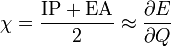$\chi = \frac{\mathrm{IP + EA} }{2} \approx \frac{\partial E}{\partial Q}$

where IP is the ionisation potential, and EA is the electron affinity. The electronic hardness is given by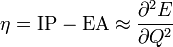$\eta = \mathrm{IP - EA} \approx \frac{\partial^2 E}{\partial Q^2}$

## Charge equilibration energy

Using the above expressions one has the following second order approximation for the total electrostatic energy ( Eq. 6)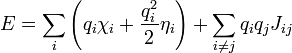$E = \sum_i \left( q_i\chi_i + \frac{q_i^2}{2} \eta_i \right) + \sum_{i \neq j} q_i q_j J_{ij}$

The last term is a "shielded" Coulombic interaction, where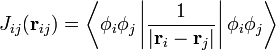$J_{ij} ({\mathbf{r}}_{ij}) = \left\langle \phi_i \phi_j \left\vert \frac{1}{| {\mathbf{r}}_{i} - {\mathbf{r}}_{j} |} \right\vert \phi_i \phi_j \right\rangle$

where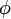$\phi$ represents a normalised ns Slater-type orbital.